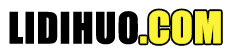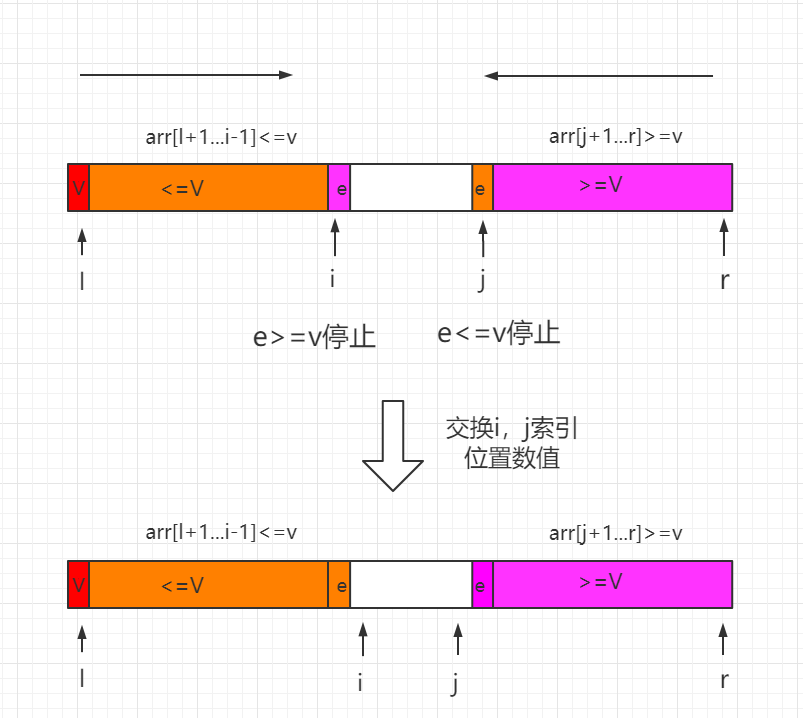# 双路快速排序

### 三、过程图示### 四、Java 实例代码

package lidihuo ;

/**
* 双路快速排序
*/

public class QuickSort2Ways {

//核心代码---开始
private static int partition ( Comparable [ ] arr, int l, int r ) {
// 随机在arr[l...r]的范围中, 选择一个数值作为标定点pivot
swap ( arr, l , ( int ) ( Math. random ( ) * (r -l + 1 ) ) +l ) ;
Comparable v = arr [l ] ;
// arr[l+1...i) <= v; arr(j...r] >= v
int i = l + 1, j = r ;
while ( true ) {
while ( i <= r && arr [i ]. compareTo (v ) < 0 )
i ++;
while ( j >= l + 1 && arr [j ]. compareTo (v ) > 0 )
j --;
if ( i > j )
break ;
swap ( arr, i, j ) ;
i ++;
j --;
}
swap (arr, l, j ) ;
return j ;
}
//核心代码---结束

// 递归使用快速排序,对arr[l...r]的范围进行排序
private static void sort ( Comparable [ ] arr, int l, int r ) {
if (l >= r ) {
return ;
}
int p = partition (arr, l, r ) ;
sort (arr, l, p - 1 ) ;
sort (arr, p + 1, r ) ;
}

public static void sort ( Comparable [ ] arr ) {

int n = arr. length ;
sort (arr, 0, n - 1 ) ;
}

private static void swap ( Object [ ] arr, int i, int j ) {
Object t = arr [i ] ;
arr [i ] = arr [j ] ;
arr [j ] = t ;
}

// 测试 QuickSort
public static void main ( String [ ] args ) {

// 双路快速排序算法也是一个O(nlogn)复杂度的算法
// 可以在1秒之内轻松处理100万数量级的数据

// Quick Sort也是一个O(nlogn)复杂度的算法
// 可以在1秒之内轻松处理100万数量级的数据
int N = 1000000 ;
Integer [ ] arr = SortTestHelper. generateRandomArray (N, 0, 100000 ) ;
sort (arr ) ;
SortTestHelper. printArray (arr ) ;

}
}